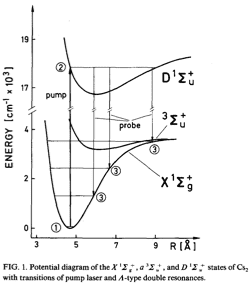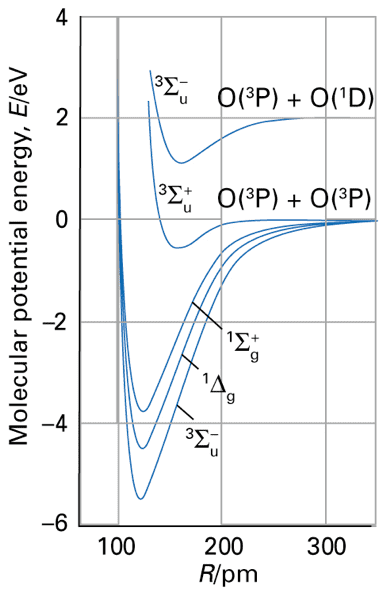# Isotope shift in molecules

• I
kelly0303
Hello! Is the isotope shift between 2 (low lying) vibrational levels of the lowest 2 electronic levels (of a diatomic molecule) the same, no matter what the 2 levels are? For example, is the isotope shift associated to the ##0 \to 0## vibrational transition of 2 molecular isotopes (here I mean the lowest vibrational level of the electronic ground state and the lowest vibrational level of the first excited electronic state) the same as the ##1 \to 1## isotope shift (here I mean the first excited vibrational level of the electronic ground state and the first excited vibrational level of the first excited electronic state)? And if they are not the same, how big (order of magnitude) is the difference? Any insight or suggested reading would be greatly appreciated. Thank you!

Mentor
As far as I remember the two energy spectra should be roughly independent. In other words:
E_total = E_electronic + E_vibration where both components have their own isotope shift. If you consider the isotope effect on a transition only then E_transition_isotope = E_electronic_transition_isotope + E_vibration_transition_isotope. As long as you don't change the vibrational state I don't expect the isotope shift to depend on that state.

Homework Helper
I think the principle is that the energies are separable, i.e. E_total = E_electronic + (n+1/2)hν (ignoring anharmonicity). But ν may, and generally does, vary from one electronic state to another, as the different electronic distribution affects the stiffness of bonds. Then
E(0→0) = ΔEe + 1/2 hΔν
E(1→1) = ΔEe + 3/2 hΔν
So E(0→0) is different from E(1→1) for the same isotopomer.
The isotope shift for E(0→0) will be 1/2 h(Δν1 - Δν2) = 1/2 hΔν1(1-μ12)
where the subscript 1 denotes the lighter isotopomer, and μ is the reduced mass, and for E(1→1) will be
3/2 hΔν1(1-μ12)

Mentor
as the different electronic distribution affects the stiffness of bonds
Then there is a difference, but that sounds like a tiny higher order effect.

Mentor
Then there is a difference, but that sounds like a tiny higher order effect.
Certainly not!

Here is an example for Cs2and this one for O2These curves definitely correspond to very different bond stiffness, and thus different ##\nu##.

•mfb
kelly0303
I think the principle is that the energies are separable, i.e. E_total = E_electronic + (n+1/2)hν (ignoring anharmonicity). But ν may, and generally does, vary from one electronic state to another, as the different electronic distribution affects the stiffness of bonds. Then
E(0→0) = ΔEe + 1/2 hΔν
E(1→1) = ΔEe + 3/2 hΔν
So E(0→0) is different from E(1→1) for the same isotopomer.
The isotope shift for E(0→0) will be 1/2 h(Δν1 - Δν2) = 1/2 hΔν1(1-μ12)
where the subscript 1 denotes the lighter isotopomer, and μ is the reduced mass, and for E(1→1) will be
3/2 hΔν1(1-μ12)
Thank you for this! Actually I should have been more clear in my question (sorry for that). I am interested in high mass isotopes, where the mass shift is basically negligible i.e. ##\mu1/\mu2## is basically 1. What I am interested in is the field (volume) effect of isotope shift. I didn't find much online so any suggested reading would be really welcome.